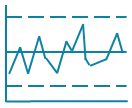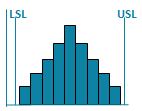# Block Diagram in Excel

## Use a Block Diagram to Design Reliability of Systems### There are three types of block diagrams:

• System - physical relationship of major system components
• Functional - Categorize system components according to the function they provide.
• Reliability - depicts the effect of a component failure on the system's function.

### To Create a Block Diagram

1. Lay out a diagram of parallel and serial components
2. Identify probability of failure of each component (value 0-1).
3. Calculate parallel probabilities. (a+b-a*b)
4. Multiply serial component probabilities (a*b)
5. Use parallel parts to increase reliability.

QI Macros add-in for Excel includes a block diagram template to get you started.

### Other charts in QI Macros add-in

Control ChartsHistogramsPareto ChartsBox PlotsFishbone Diagram